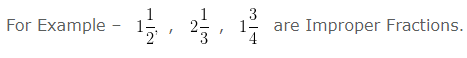# Proper Fraction | Improper Fraction | Mixed Fraction | Maths

Proper Fraction

Proper Fraction is a Fraction whose numerator is less than its denominator.

i.e Numerator < Denominator

For Example – 1/3, 3/7, 5/6 are Proper Fractions.

Improper Fraction

Improper Fraction is a Fraction whose numerator is greater than or equal to its denominator.

i.e Numerator ≥ Denominator

For Example – 3/2, 7/4, 6/5 are Improper Fractions.

Mixed Fraction

Mixed Fraction is a Fraction having combination of a Whole number or a Proper Fraction.QUESTION

Is 3/2 is an Improper Fraction ?

EXPLANATION

In the given Fraction, Numerator = 3
Denominator = 2
Here, 3 > 2
i.e, Numerator > Denominator
Hence, 3/2 is an Improper Fraction

QUESTION

Is 5/7 is an Improper Fraction?

EXPLANATION

In the given Fraction, Numerator = 5
Denominator = 7
Here, 5 < 7
i.e, Numerator < Denominator
Hence, 5/7 is not an Improper Fraction

QUESTION

Is 5/9 is a Proper Fraction ?

EXPLANATION

In the given Fraction, Numerator = 5
Denominator = 9
Here, 5 < 9
i.e, Numerator < Denominator
Hence, 5/9 is a Proper Fraction

QUESTION

Is 4/3 is a Proper Fraction ?

EXPLANATION

In the given Fraction, Numerator = 4
Denominator = 3
Here, 4 > 3
i.e, Numerator > Denominator
Hence, 4/3 is not a Proper Fraction